﻿

### Ideal properties and integral extension of convolution operators on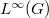$L^\infty (G)$

#### Abstract

We investigate operator ideal properties of convolution operators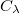$C_\lambda$ (via measures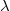$\lambda$) acting in${L^\infty (G)}$, with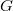$G$ a compact abelian group. Of interest is when$C_\lambda$ is compact, as this corresponds to$\lambda$ having an integrable density relative to Haar measure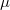$\mu$, i.e.,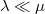$\lambda \ll \mu$. Precisely then is there an \textit{optimal} Banach function space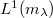$L^1 (m_\lambda)$ available which contains${L^\infty (G)}$ properly, densely and continuously and such that$C_\lambda$ has a continuous,${L^\infty (G)}$-valued, linear extension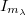$I_{m_\lambda}$ to$L^1 (m_\lambda)$. A detailed study is made of$L^1 (m_\lambda)$ and$I_{m_\lambda}$. Amongst other things, it is shown that$C_\lambda$ is compact iff the finitely additive,${L^\infty (G)}$-valued set function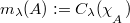$m_\lambda (A) := C_\lambda ({\chi_{_{_{\scriptstyle{A}}}}})$ is norm$\sigma$-additive iff$\lambda \in L^1 (G)$, whereas the corresponding optimal extension$I_{m_\lambda}$ is compact iff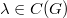$\lambda \in C (G)$ iff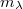$m_\lambda$ has finite variation. We also characterize when$m_\lambda$ admits a Bochner (resp.\ Pettis)$\mu$-integrable,$L^{\infty} (G)$-valued density.

DOI Code: 10.1285/i15900932v31n1p149

Keywords: Convolution operator ; vector measure ; optimal domain ; Bochner-Pettis density

Full Text: PDFThis work is licensed under a Creative Commons Attribuzione - Non commerciale - Non opere derivate 3.0 Italia License.